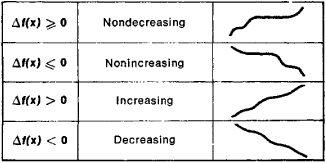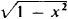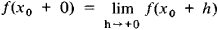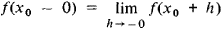# Monotonic Function

(redirected from Antitone)

## monotonic function

[¦män·ə¦tän·ik ′fəŋk·shən]
(mathematics)

## Monotonic Function

(or monotone function), a function whose increments Δf(x) = f(x′) − f(x) do not change sign when Δx = x′ − x > 0; that is, the increments are either always nonnegative or always nonpositive. Somewhat inaccurately, a monotonic function can be defined as a function that always varies in the same direction. Different types of monotonic functions are represented in Figure 1. For example, the function y = x3 is an increasing function. If a function f(x) has a derivative f′(x) that is nonnegative at every point and that vanishes only at a finite number of individual points, then f(x) is an increasing function. Similarly, if f′(x) ≤ 0 and vanishes only at a finite number of points, then f(x) is a decreasing function.Figure 1

A monotonicity condition can hold either for all x or for x on a given interval. In the latter case, the function is said to be monotonic on this interval. For example, the function y =increases on the interval [−1,0] and decreases on the interval [0, +1]. A monotonic function is one of the simplest classes of functions and is continually encountered in mathematical analysis and the theory of functions. If f(x) is a monotonic function, then the following limits exist for any X0:andReferences in periodicals archive ?
The antitone sequence UB curve was drawn in the same way.
(2) [??]: P x P [right arrow] P is isotone on first variable and antitone on second variable.
Note that the pair of operators ([up arrow], [down arrow]) forms an antitone Galois connection, while the pairs of operators ([??],[??]) and ([??], [??]) formisotone Galois connections .More properties about these operators can be found below.
Another advantage of this method is that the operator in the finite element problem is diagonally isotone and off-diagonally antitone and thereby some monotone convergent algorithms can be applied (see, e.g., [13, 14]).
Generally, the operator [F.sub.h]([mu]) in problem (10) is not diagonally isotone and off-diagonally antitone. Moreover, from the computational point of view, it is tedious to calculate the nonsmooth term [MATHEMATICAL EXPRESSION NOT REPRODUCIBLE IN ASCII] directly and some numerical quadrature algorithms have to be used.
Furthermore, it will be seen in Section 3 that the operator in problem (11) is a diagonally isotone and off-diagonally antitone operator if we assume that the triangulation satisfied the so-called maximum angle condition.
Now, define an antitone S = ([S.sub.r] | r [member of] Q) [subset or equal to] S(L) as follows:
This method is in some manner the most general one and it covers most of the mentioned approaches based on the antitone Galois connections.
Since the theory of concept lattices is closely related to antitone Galois connections and closure systems, we give a brief overview of these notions in the preliminary section.
In this section we mention some results concerning the antitone Galois connections and their relationship with the closure systems in complete lattices.
3.2 Definition A set of possible worlds, seen as a function f: [2.sup.n] [approaches] 2 is called negative or antitone if f reverses the order.
Therefore, in order to achieve the expansion of the real number [[phi].sub.m], one approach is to build antitone (reverse) grey prediction models of sequences [X.sub.a] and [X.sub.b], so that the process to simulate the limit [a.sub.1] and lower limit [[b.sub.1] of interval grey number [cross product]([t.sub.1]) could be conducted.

Site: Follow: Share:
Open / Close